### AME 301: Differential Equations, Vibrations and Control Homework 1

 B. GoodwineJ. Lucey Fall, 2003 Issued: August 29, 2003 Due: September 3, 2003

For each of the following equations, identify them as

• linear or nonlinear (2 points),
• homogeneous or inhomogeneous (1 point),
• variable or constant coefficients (1 point), and
• ordinary or partial (1 point).

If the differential equation is a linear, homogeneous, constant coefficient ordinary differential equation, determine its solution (5 points).

1.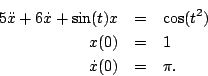2.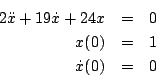3.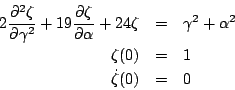4.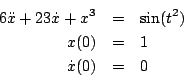5.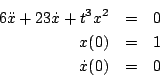6.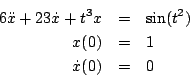7.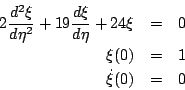8.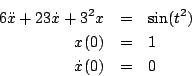9.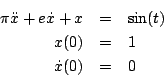10.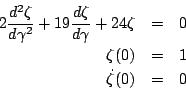11.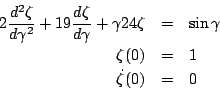Last updated: August 29, 2003.
B. Goodwine (goodwine@controls.ame.nd.edu)